Ferrari F-355 Aerodynamics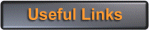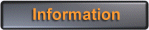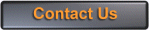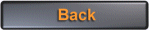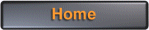We were watching a motor race a few years ago involving a Ferrari F-355 which was running with its retractable headlights open. The race was taking place on a high speed circuit, so we were curious to determine what effect this had on the vehicle performance.

This analysis is an approximate drag prediction for a Ferrari F-355, in order to determine the effect of this headlight drag.

The following data was obtained for the F-355 from various sources;

 Car Width Car Height Coefficient of Drag Engine Power Maximum Speed 77.5 inches 44.5 inches 0.35 375 horsepower 295 kmh (184 mph or 270 fps)

First we need to calculate a few required values. The frontal area of the car is;

S = (77.5 x 44.5) / 144
S = 23.95 square feet

We'll call this 24 square feet. All this analysis will take place at the maximum speed of the vehicle, using Imperial units. The dynamic pressure at the maximum vehicle speed is;

Q = 0.5 x rho x V x V

where rho = air density = 0.002378 slugs per cubic foot, so,

Q = 0.5 x 0.002378 x (270 x 270)
Q = 86.68 pounds per square foot

Now we need to determine the total drag of the vehicle at its maximum speed.

D = Cd x S x Q
D = 0.35 x 24.0 x 86.68 = 728 pounds

We need to make an estimate of the drag of the open headlights. We'll assume the headlights have a physical size of about 8 inches by 10 inches each. This is then,

(8.0 x 10.0) / 144 = 0.55 square foot per headlight

We can assume
Cd = 1.0 for the flat vertical surface of each light, so the drag is;

D = Cd x S x Q D = 1.0 x 0.55 x 86.68 per light
D = 47.7 pounds per light, or 95.3 pounds in total

We can now calculate a new top speed due to this slight increase in drag. The drag of a car varies as the square of its velocity. Taking proportions;

(D2 / D1) = (V2 x V2) / (V1 x V1)
V2 = sqrt(( 270 x 270) x (728 / (728 + 95.3))
V2 = 253.8 feet per second

So the maximum vehicle speed with lights open is 254 fps, compared to 270 fps with the lights closed. The open lights result in a degradation in maximum speed of 16 feet per second (10.9 mph).

Finally, just to do a bit of a check on this analysis, estimating the vehicle drag based on engine power gives the following result;

D = (550 x P ) / V
D = (550 x 375 x 0.95) / 270
D = 725.7 pounds
(compared to 728 pounds determined previously)

Note that we take the 375 horsepower maximum engine power here and apply a 0.95 factor to it, to allow for drivetrain losses.

Finally, we can check the drag coefficient;

Cd x S = (2 x D) / (rho x V x V)
Cd x S = (2 x 725.7) / (0.002378 x 270 x 270)
Cd x S = 8.37 square feet

So,

Cd = 8.37 / 24.0 = 0.349
(compared to 0.35 from independent sources)

 Copyright (C) 2018 Temporal Images Email Us Last Updated: 1st November 2018